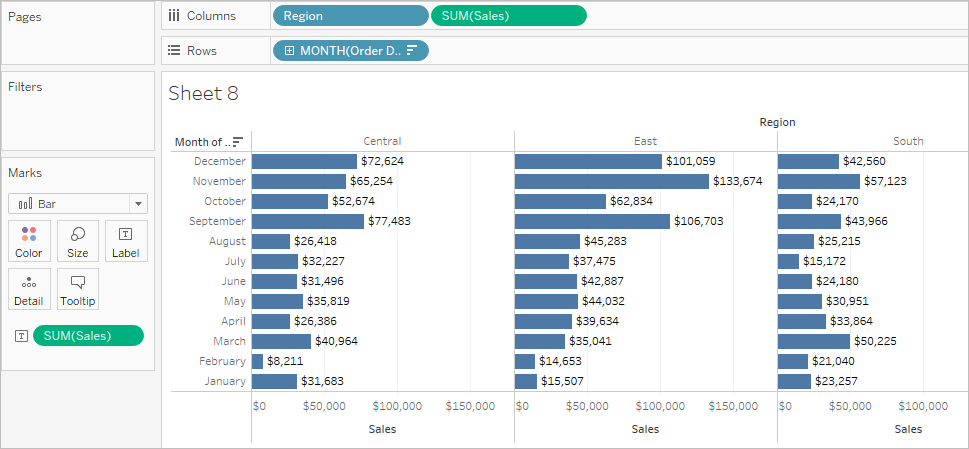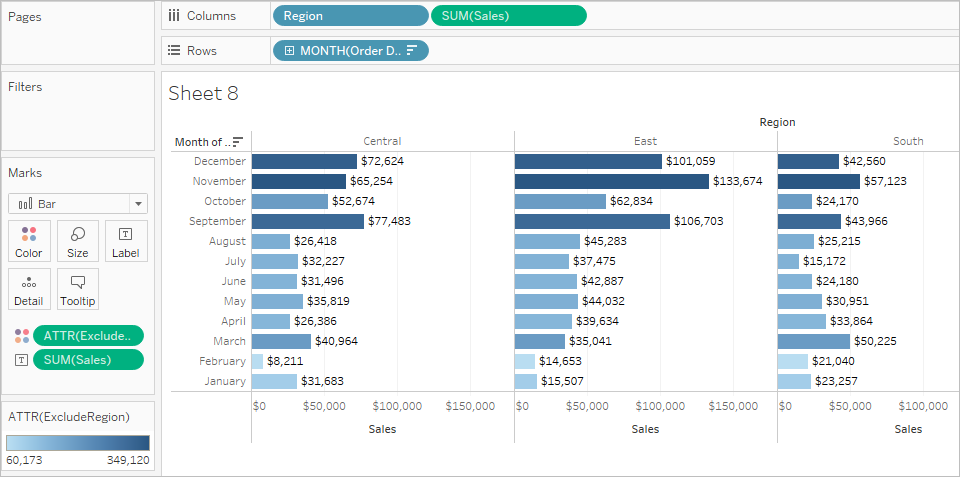# EXCLUDE Level of Detail Expressions

EXCLUDE level of detail expressions omit declared dimensions from the view.

EXCLUDE level of detail expressions are useful for ‘percent of total’ or ‘difference from overall average’ scenarios. They are comparable to such features as Totals and Reference Lines.

EXCLUDE level of detail expression cannot be used in row-level expressions (where there are no dimensions to omit), but can be used to modify either a view level calculation or anything in between (that is, you can use an EXCLUDE calculation to remove dimension from some other level of detail expression).

## Example 1

The following level of detail expression excludes [Region] from a calculation of the sum of [Sales]:

`{EXCLUDE [Region]: SUM([Sales])}`

The expression is saved as [ExcludeRegion].

To illustrate how this expression might be useful, first consider the following view, which breaks out the sum of sales by region and by month:Dropping [ExcludeRegion] on Color shades the view to show total sales by month but without the regional component:## Example 2

The following EXCLUDE level of detail expression computes the average sales total per month and then excludes the month component:

`{EXCLUDE [Order Date (Month / Year)] : AVG({FIXED [Order Date (Month / Year)] : SUM([Sales])})}`

Notice that this is a nested level of detail expression—that is, a level of detail expression within another level of detail expression.

Saved as [average of sales by month], the calculation can then be subtracted from the sum of sales per month by means of an ad-hoc calculation on the Rows shelf:With Month([Order Date]) on the Columns shelf, this creates a view that shows the difference between actual sales per month over a four-year period and the average monthly sales for the entire four-year period: